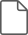Hostname: page-component-7d8f8d645b-h5t26 Total loading time: 0 Render date: 2023-05-29T00:17:35.502Z Has data issue: false Feature Flags: { "useRatesEcommerce": true } hasContentIssue false

## Abstract

HTML view is not available for this content. However, as you have access to this content, a full PDF is available via the ‘Save PDF’ action button.

Consider two widely used definitions of equality. That of Leibniz: one value equals another if any predicate that holds of the first holds of the second. And that of Martin-Löf: the type identifying one value with another is occupied if the two values are identical. The former dates back several centuries, while the latter is widely used in proof systems such as Agda and Coq. Here we show that the two definitions are isomorphic: we can convert any proof of Leibniz equality to one of Martin-Löf identity and vice versa, and each conversion followed by the other is the identity. One direction of the isomorphism depends crucially on values of the type corresponding to Leibniz equality satisfying functional extensionality and Reynolds’ notion of parametricity. The existence of the conversions is widely known (meaning that if one can prove one equality then one can prove the other), but that the two conversions form an isomorphism (internally) in the presence of parametricity and functional extensionality is, we believe, new. Our result is a special case of a more general relation that holds between inductive families and their Church encodings. Our proofs are given inside type theory, rather than meta-theoretically. Our paper is a literate Agda script.

Type
Theoretical Pearl
Information

## References

Altenkirch, T., McBride, C. & Swierstra, W. (2007) Observational equality, Now! In Workshop on Programming Languages Meets Program Verification. ACM, pp. 5768.Google Scholar
Atkey, R., Ghani, N. & Johann, P. (2014) A relationally parametric model of dependent type theory. In Principles of Programming Languages (POPL). ACM, pp. 503515.CrossRefGoogle Scholar
Bernardy, J.-P., Coquand, T. & Moulin, G. (2015) A Presheaf model of parametric type theory. Electr.Notes Theor. Comput. Sci. 319(Supplement C), 6782.CrossRefGoogle Scholar
Bernardy, J.-P., Jansson, P. & Paterson, R. (2010) Parametricity and dependent types. In International Conference on Functional Programming (ICFP). ACM, pp. 345356.CrossRefGoogle Scholar
Bernardy, J.-P., Jansson, P. & Paterson, R. (2012) Proofs for free—parametricity for dependent types. J. Funct. Program. 22(2), 107152.CrossRefGoogle Scholar
Cavallo, E. & Harper, R. 2019. Parametric Cubical Type Theory. arXiV:1901.00489.Google Scholar
Cockx, J. & Devriese, D. (2017) Lifting proof-relevant unification to higher dimensions. In Certified Programs and Proofs (CPP). ACM, pp. 173181.CrossRefGoogle Scholar
Cockx, J., Devriese, D. & Piessens, F. (2014) Pattern matching without K. In International Conference on Functional Programming (ICFP). ACM.CrossRefGoogle Scholar
Cockx, J., Devriese, D. & Piessens, F. (2016) Unifiers as equivalences: Proof-relevant unification of dependently typed data. In International Conference on Functional Programming (ICFP). ACM, pp. 270283.CrossRefGoogle Scholar
Cohen, C., Coquand, T., Huber, S. & Mörtberg, A. (2016) Cubical Type Theory: A constructive interpretation of the univalence axiom. Arxiv e-prints.Google Scholar
Developers, Coq. (2017) Coq documentation.Google Scholar
Coquand, T. (1992) Pattern matching with dependent types. In Types for Proofs and Programs. TYPES.Google Scholar
Hasegawa, R. (1994) Categorical data types in parametric polymorphism. Math. Struct. Comput. Sci. 4(1), 71109.CrossRefGoogle Scholar
Hofmann, M. & Streicher, T. (1996) The groupoid interpretation of type theory. In Venice Festschrift. Oxford University Press, pp. 83111.Google Scholar
Leibniz, G. (1686) Discourse on metaphysics.Google Scholar
Martin-Löf, P. (1975) An intuitionistic theory of types: Predicative part. In Logic Colloquium. Studies in Logic and the Foundations of Mathematics, vol. 80. Elsevier, pp. 73118.CrossRefGoogle Scholar
Nuyts, A. & Devriese, D. (2018) Degrees of relatedness: A unified framework for parametricity, irrelevance, Ad Hoc polymorphism, intersections, unions and algebra in dependent type theory. In Symposium on Logic in Computer Science (LICS). ACM.CrossRefGoogle Scholar
Nuyts, A., Vezzosi, A. & Devriese, D. (2017) Parametric quantifiers for dependent type theory. Proc. ACM Program. Lang. (PACMPL), 1(ICFP).CrossRefGoogle Scholar
Pfenning, F. & Paulin-Mohring, C. (1989) Inductively defined types in the calculus of constructions. Lecture Notes in Computer Science. Springer, New York, NY.CrossRefGoogle Scholar
Reynolds, J. C. (1983) Types, abstraction, and parametric polymorphism. In Information Processing, Mason, R. E. A. (ed), North Holland, pp. 513523.Google Scholar
Streicher, T. (1993) Investigations into Intensional Type Theory. Habilitation thesis, Ludwig-Maximilians-University Munich.Google Scholar
Univalent Foundations Program, The. (2013) Homotopy Type Theory: Univalent Foundations of Mathematics. Institute for Advanced Study: https://homotopytypetheory.org/book.Google Scholar
Voevodsky, V. (2011) Resizing Rules – Their Use and Semantic Justification. Talk at the Institute for Advanced Study in Princeton, NJ.Google Scholar